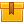## Prerequisites

This curriculum is designed with no prior knowledge required.

## Description

This library consists of eight lessons. The lessons in this library present general information about electrical schematics and electrical diagrams showing and explaining how to read and interpret the symbols on an electrical schematics and electrical diagrams.

## Objectives

Read and interpret the symbols on an electrical schematics and electrical diagrams. Learn input, logic and output devices, electrical schematics and building and line diagrams.

## ContentThis is the second lesson in the iKNOW™ Electrical Print Reading
Library. The lesson presents the symbols for various manually and
process actuated input devices and how they are represented on an
electrical schematic.

•Electrical Schematic Symbols- Input DevicesThis is the third lesson in the iKNOW™ Electrical Print Reading Library.
This lesson defines the function of logic and output elements of a
control circuit and presents the symbols for various logic and output
devices.

•Logic and Output DevicesThis is the fourth lesson in the iKNOW™ Electrical Print Reading
Library. This lesson describes the steps for interpreting the
relationships among the input, logic, and output components of an
electrical schematic.

•Interpreting Electrical SchematicsThis is the fifth lesson in the iKNOW™ Electrical Print Reading Library,
and the first lesson covering electrical diagrams. This lesson presents
information about the purpose of various types of electrical diagrams
and how to interpret the information in the title block. It also
explains how to make electrical drawing revisions.

•Introduction to Electrical DiagramsThis is the sixth lesson in the iKNOW™ Electrical Print Reading Library.
This lesson presents the different views used in electrical diagrams as
well as how to identify components, cables, and conduits. The cable
chart is also presented.

•Building Electrical DiagramsThis is the seventh lesson in the iKNOW™ Electrical Print Reading
Library. This lesson presents information regarding how to identify
loads, equipment, and isolation breakers on a single-line electrical
diagram.

•Single Line Electrical DiagramsThis is the final lesson in the iKNOW™ Electrical Print Reading Library.
The lesson presents information how to identify components, equipment,
wires and cables on a wiring diagram. It also explains how to relate a
wiring diagram to the installed hardware and how to use diagrams for
maintenance and troubleshooting problems.

•Wiring Diagrams

0
0 Reviews